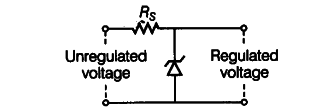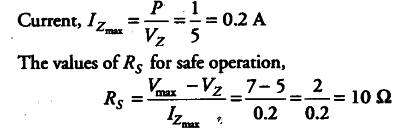# A Zener of power rating 1 W is to be used as a voltage regulator

A Zener of power rating 1 W is to be used as a voltage regulator. If Zener has a breakdown of 5 V and it has to regulate voltage which fluctuated between 3 V and 7 V, what should be the value of { R }_{ s } for safe operation as shown below figure?Given power = 1 W
Zener breakdown { V}_{z } = 5V
Minimum voltage { V}_{min } = 3V
Maximum Voltage { V}_{max} = 7V